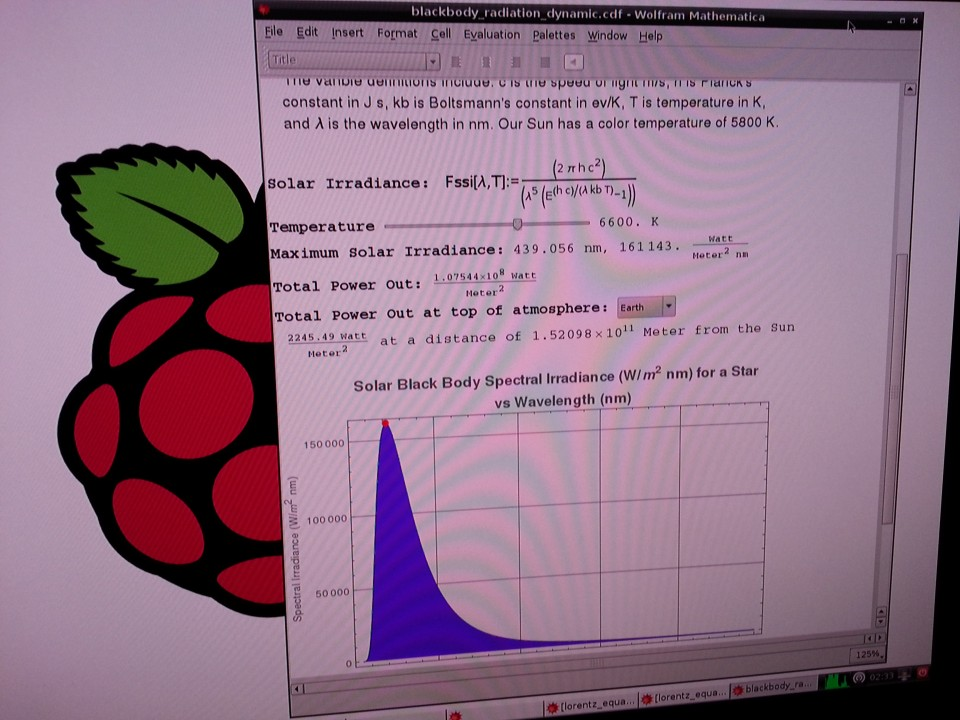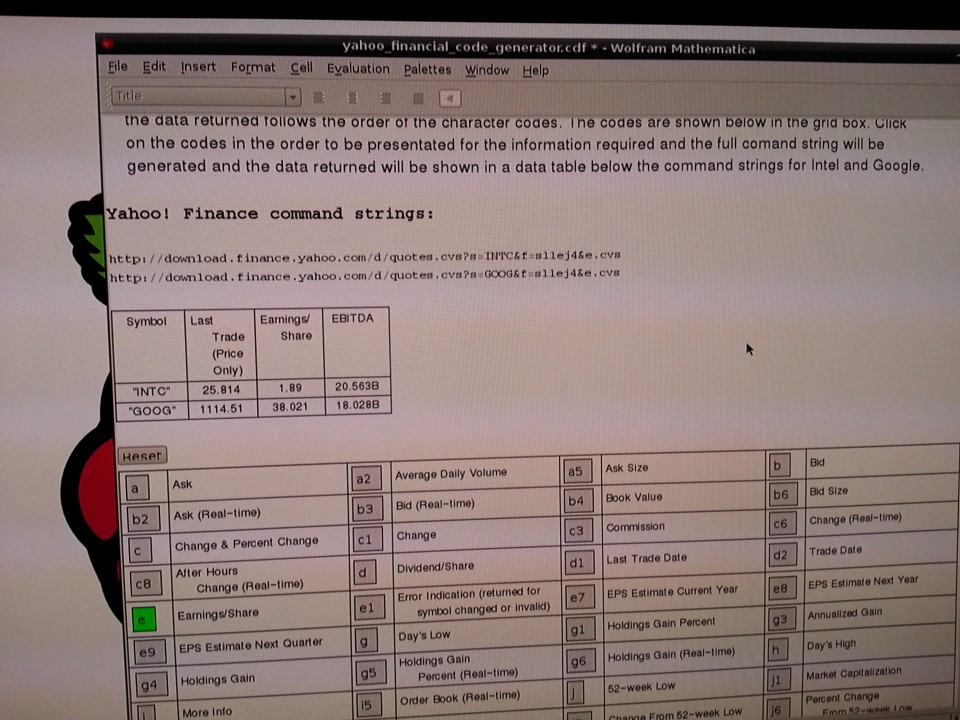# Raspberry Pi Mathematica Notebook and CDF test

I wondered if the five CDFs and their associated notebooks posted to date on this blog would run unmodified on Mathematica running on the Raspberry Pi. The answer is mostly yes. All but one ran. The Yahoo! Financial Code Generator CDF ran but the notebook did not. This notebook crashed Mathematica on the Raspberry Pi. To be fair, however, the Yahoo! Financial Code Generator needs some work. It runs continuously and should only update once a code button is selected. All version were very slow as well. This means that careful and efficient code is require for responsive operation on the Raspberry Pi.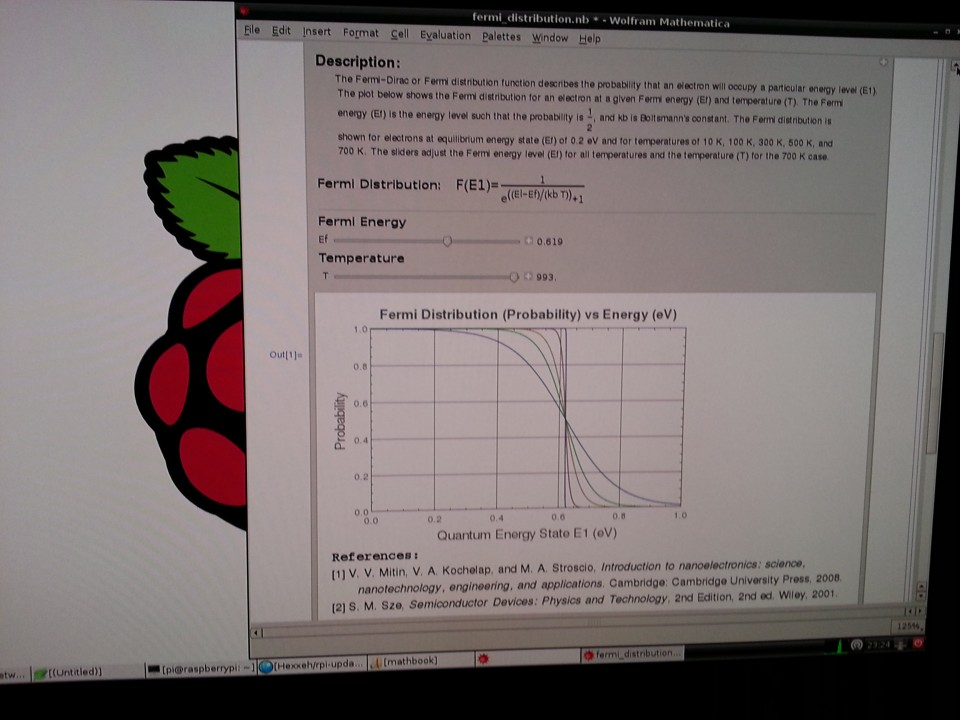Fermi Distribution Notebook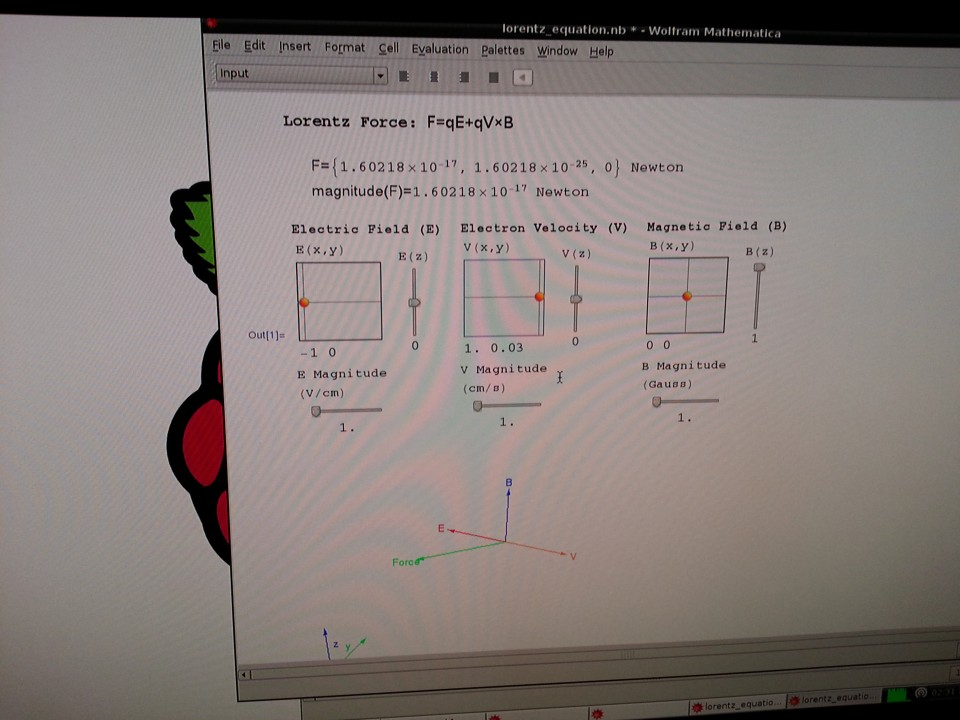Lorentz Equation Notebook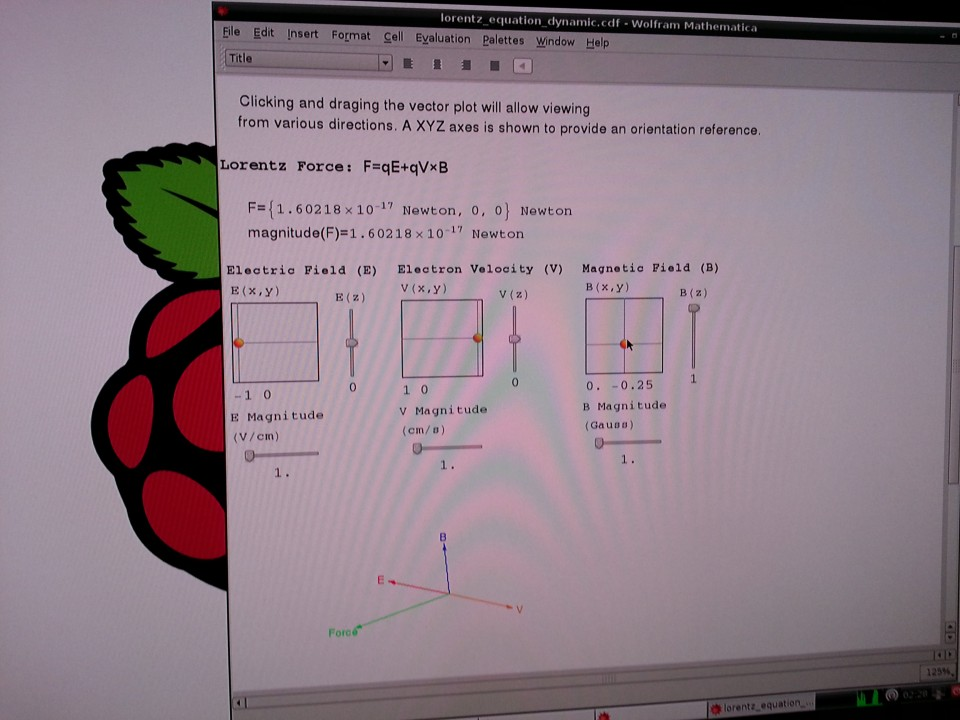Lorentz Equation CDF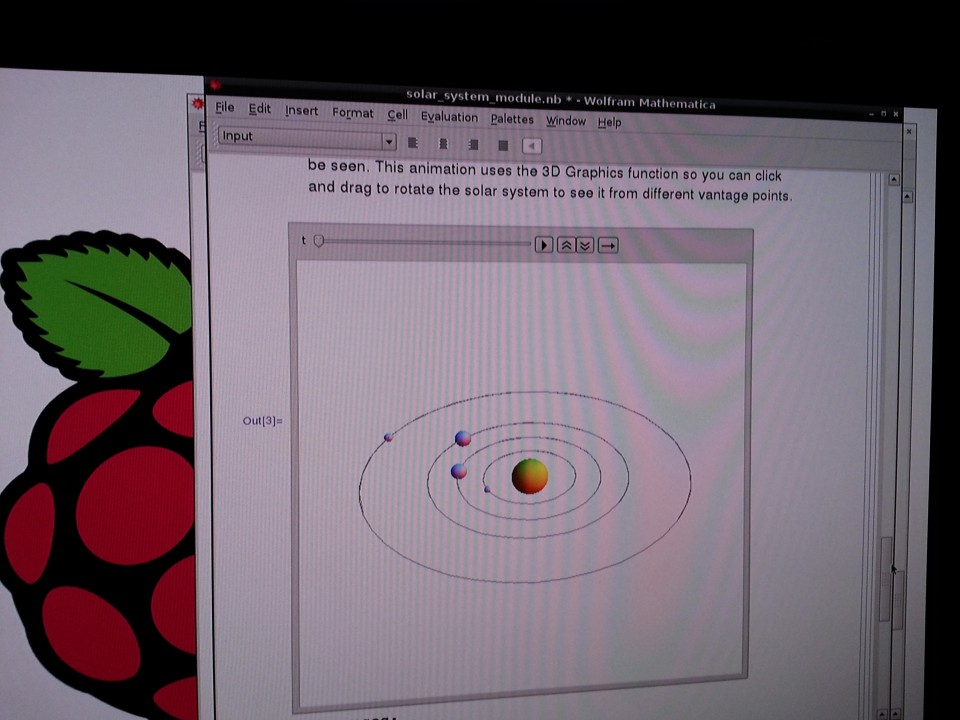Solar System Animation NotebookSolar System Animation CDF